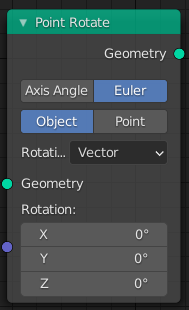# Point Rotate¶The Point Rotate node.

The Point Rotate node rotates every point of the geometry. It does so by modifying the rotation attribute.

## Inputs¶

Geometry

Standard geometry input.

Rotation

Specifies how much the point is rotate around each axis. This input is only available when the rotation type is set to Euler.

Axis

The axis to rotate the point around. This input is only available when the rotation type is set to Axis Angle.

Angle

The angle to rotate by around the specified axis. This input is only available when the rotation type is set to Axis Angle.

## Properties¶

Rotation Type
Axis Angle

Use separate axis and angle inputs to control the rotation.

Euler

Use an Euler input to control the rotation.

Space
Object

Rotate every point in the local space of the object that is being evaluated. The same space is used for every point.

Point

Rotate every point in its local space as specified by its rotation attribute.

## Outputs¶

Geometry

Standard geometry output.